# RS Aggarwal Solutions for Class 12 Maths Chapter 12: Indefinite Integral

We know that the inverse process of differentiation is integration. This exercise covers the concept of integration and important formulas, which are used in solving the problems. The solutions are designed by highly experienced faculty in a stepwise manner as per the exam pattern of the current board. This improves the analytical abilities among students which help them in their exam preparation. In order to score good marks in the board exams, students can access RS Aggarwal Solutions for Class 12 Maths Chapter 12 Indefinite Integral free PDF, from the links which are given below.

## RS Aggarwal Solutions for Class 12 Maths Chapter 12: Indefinite Integral Download PDF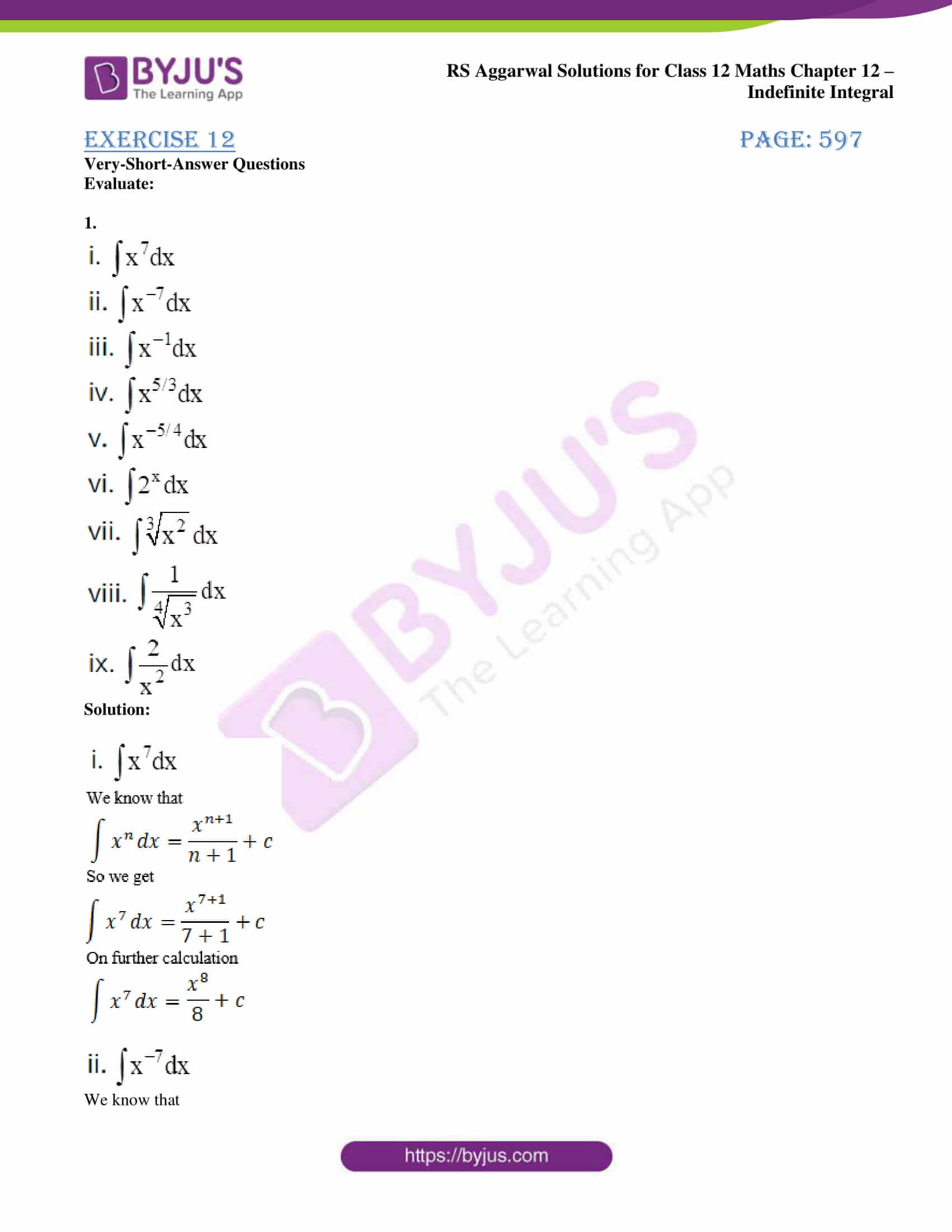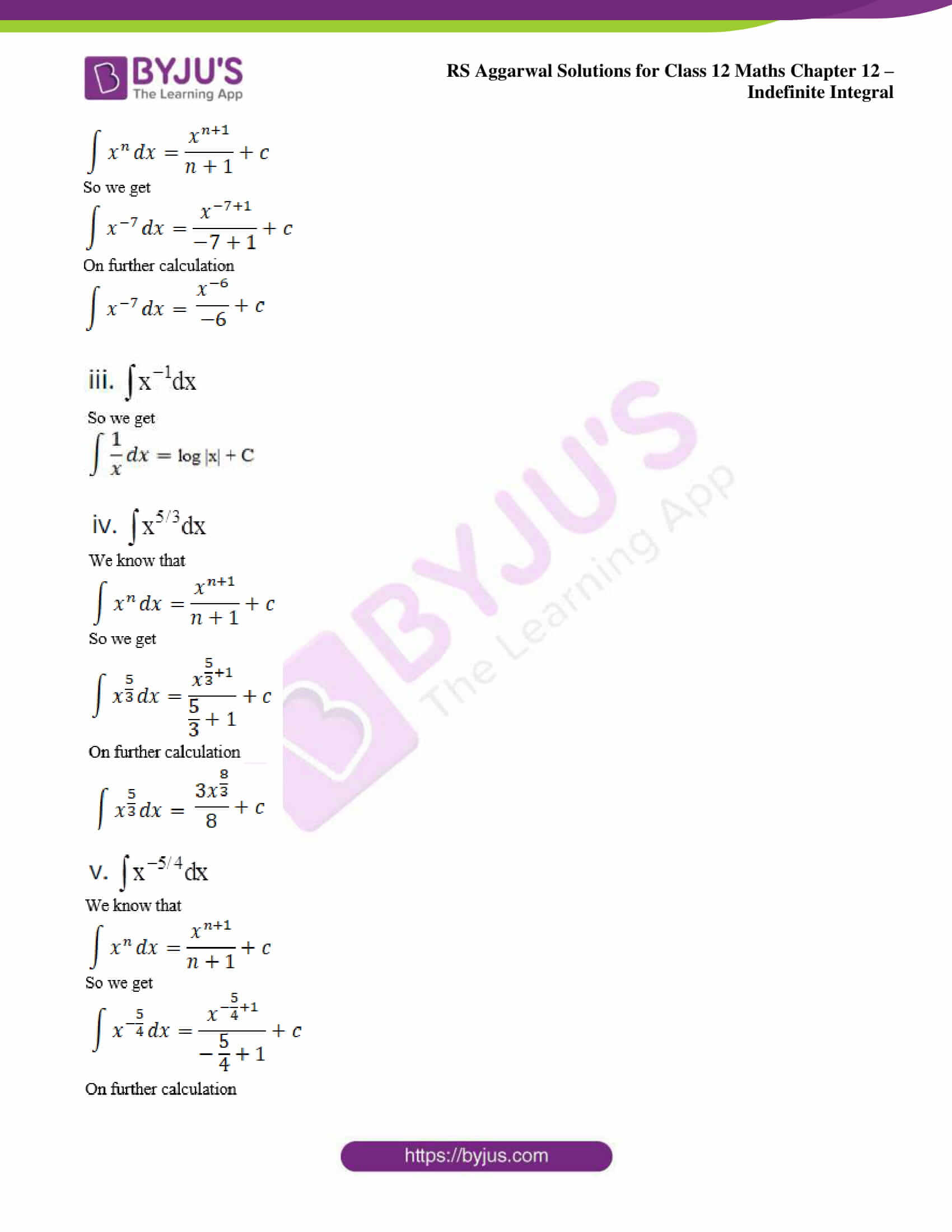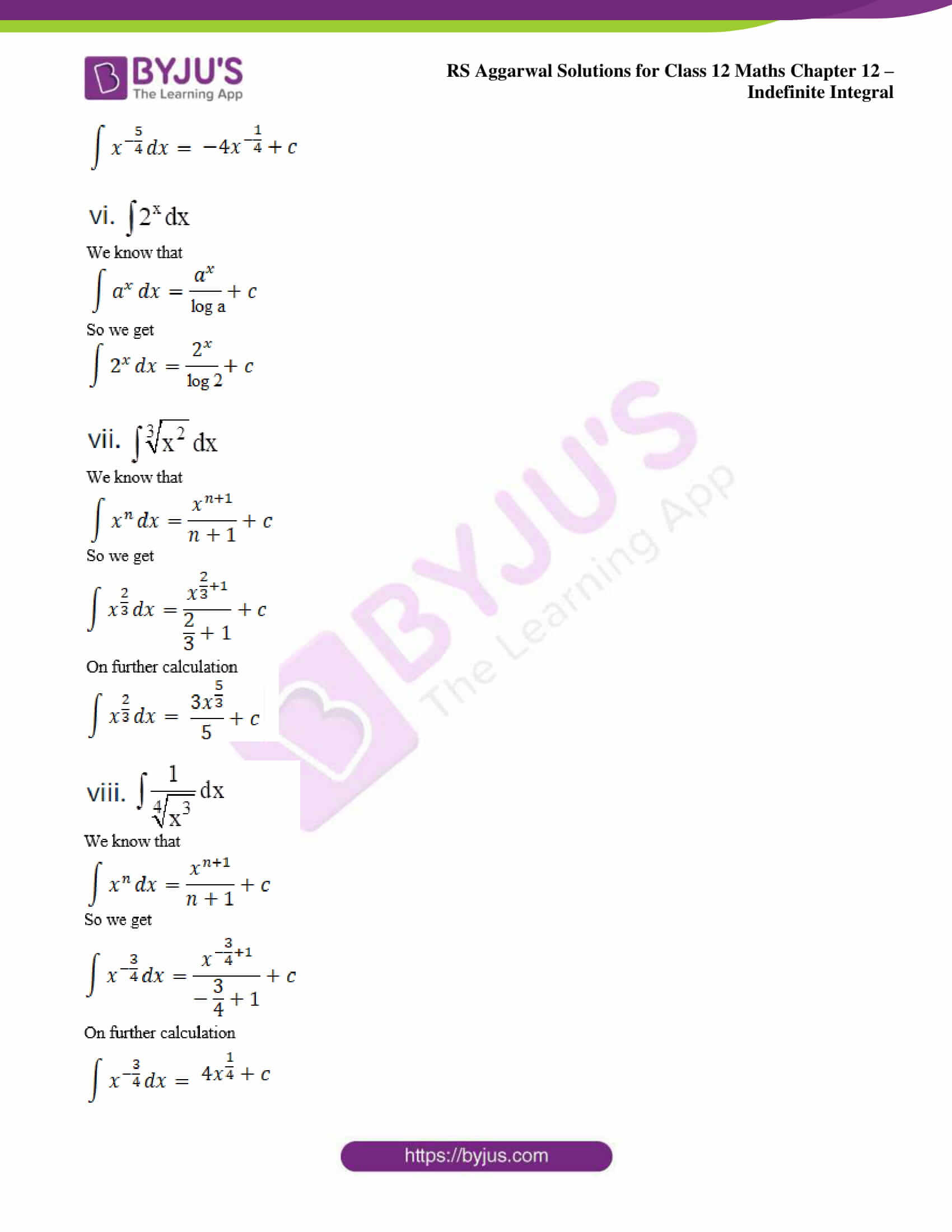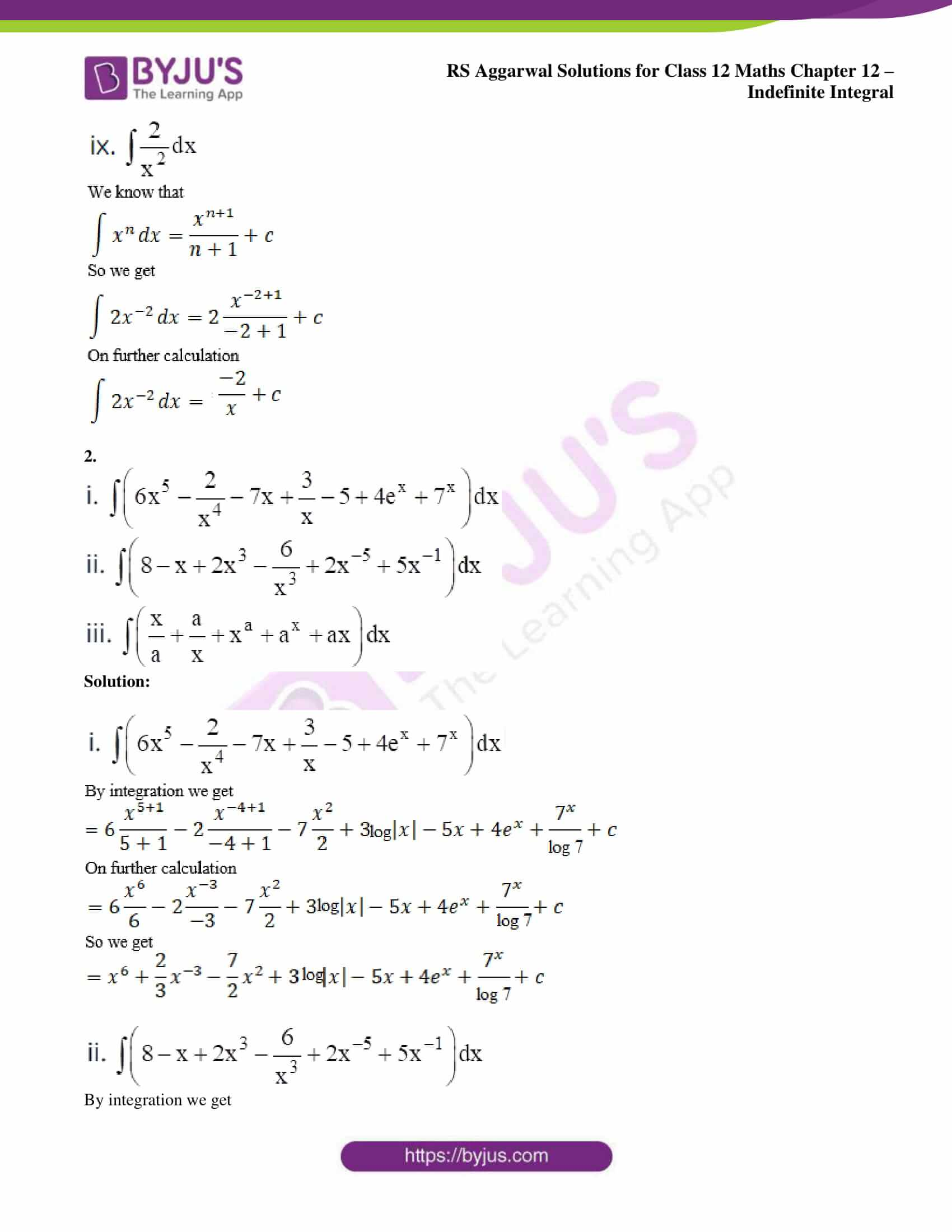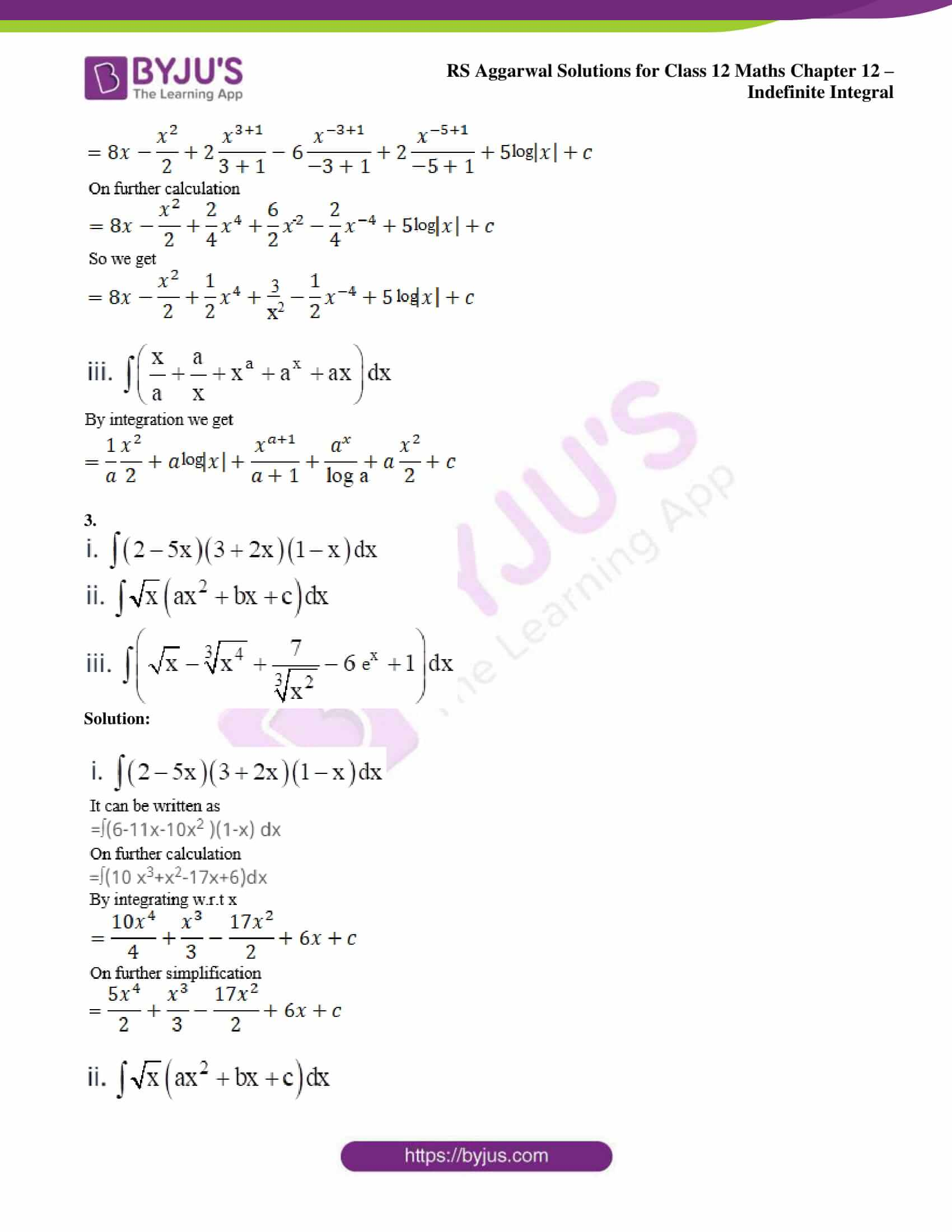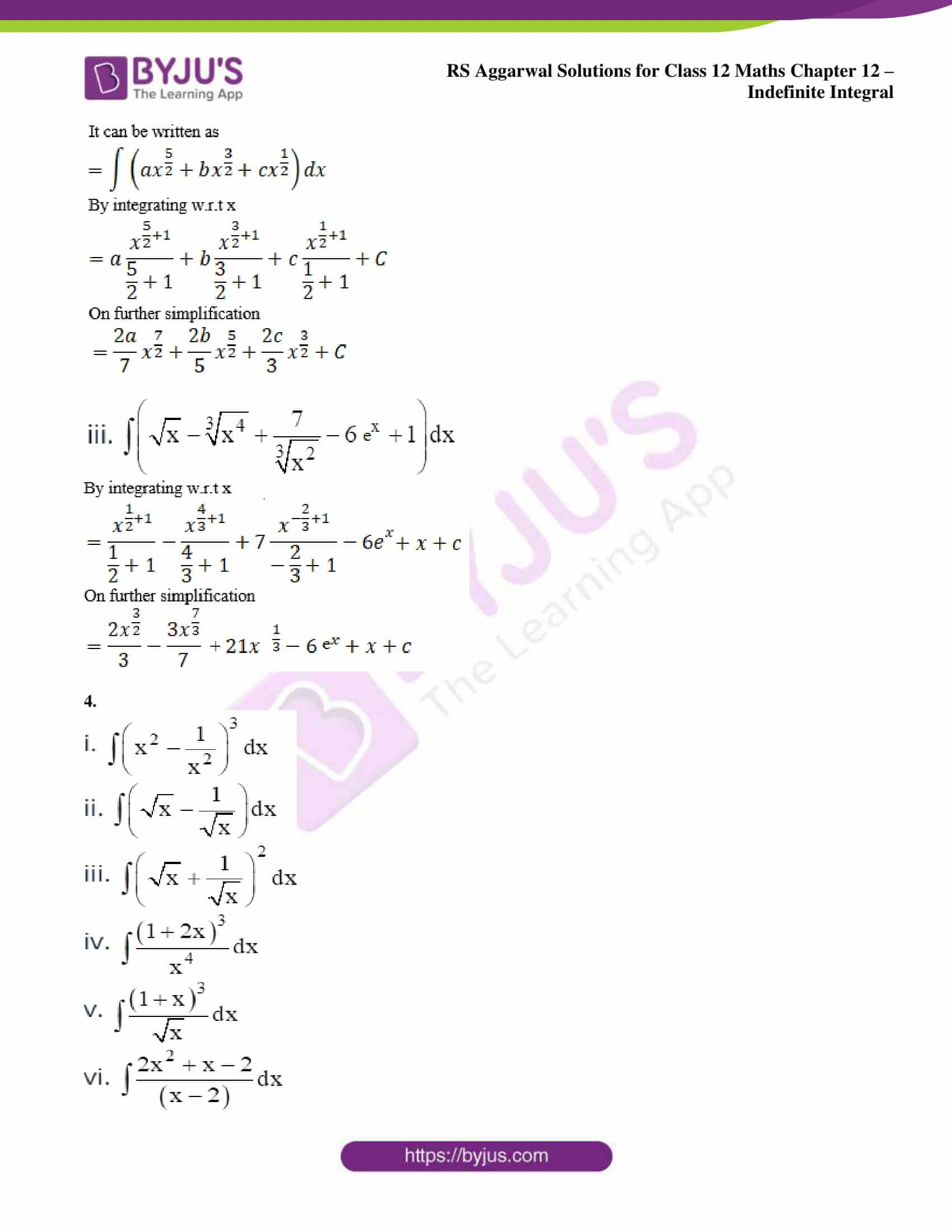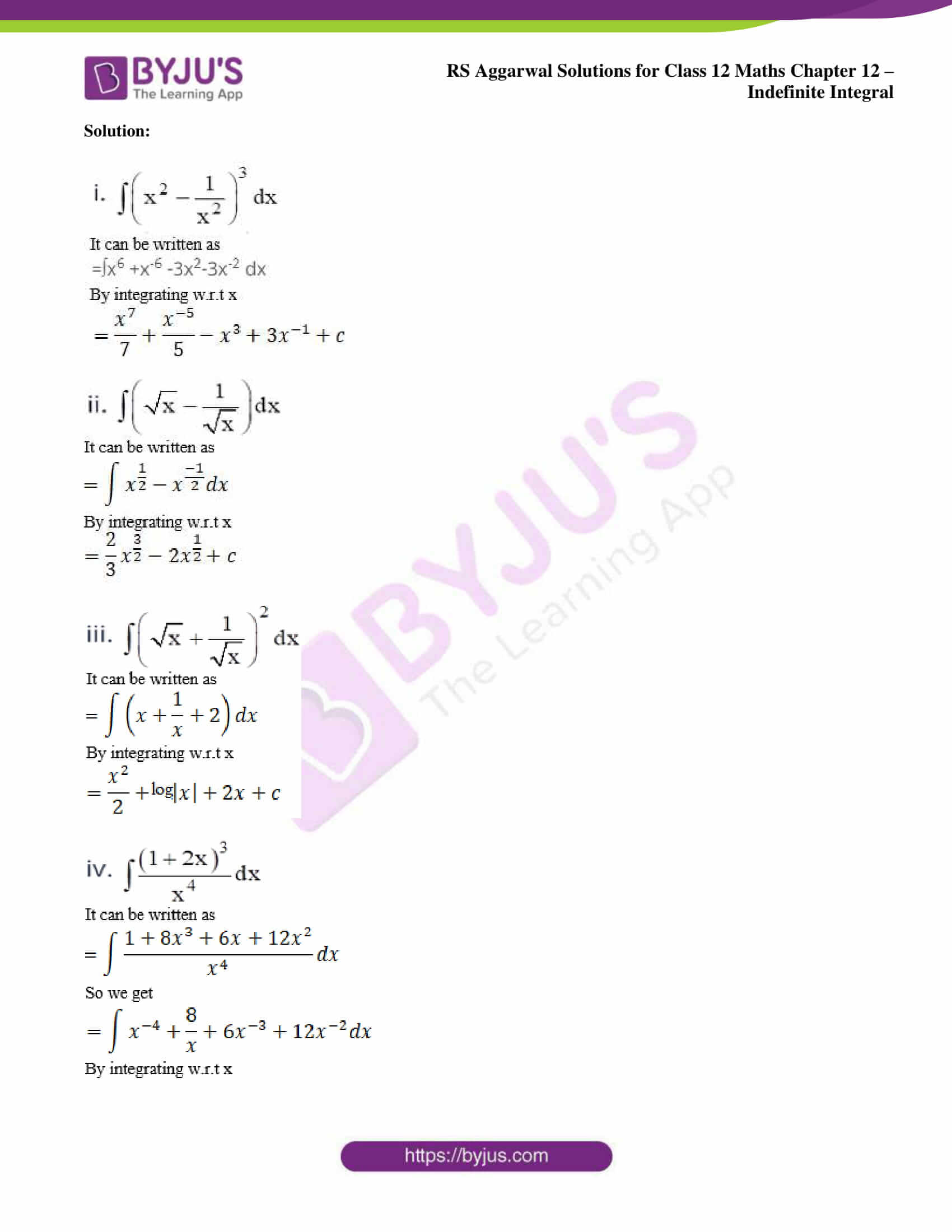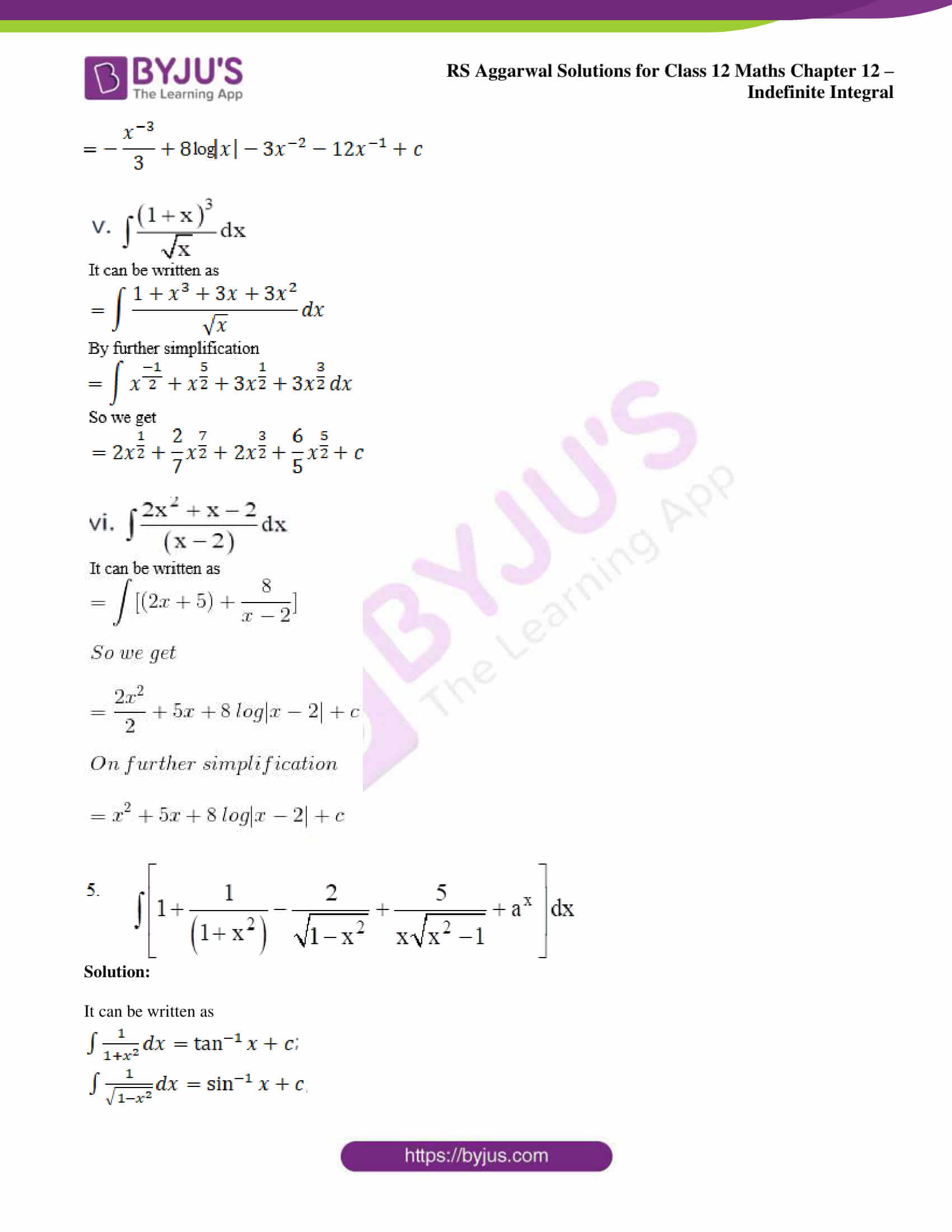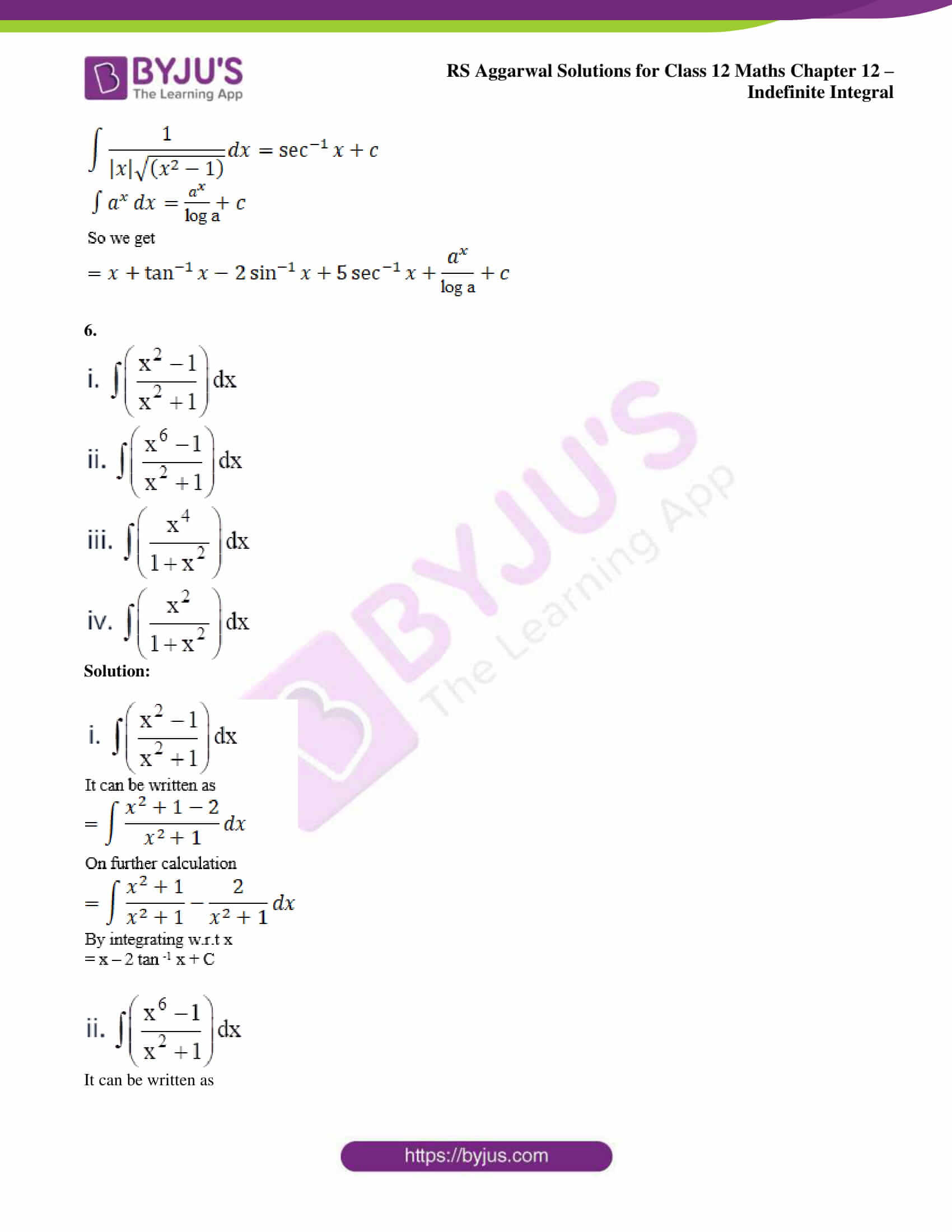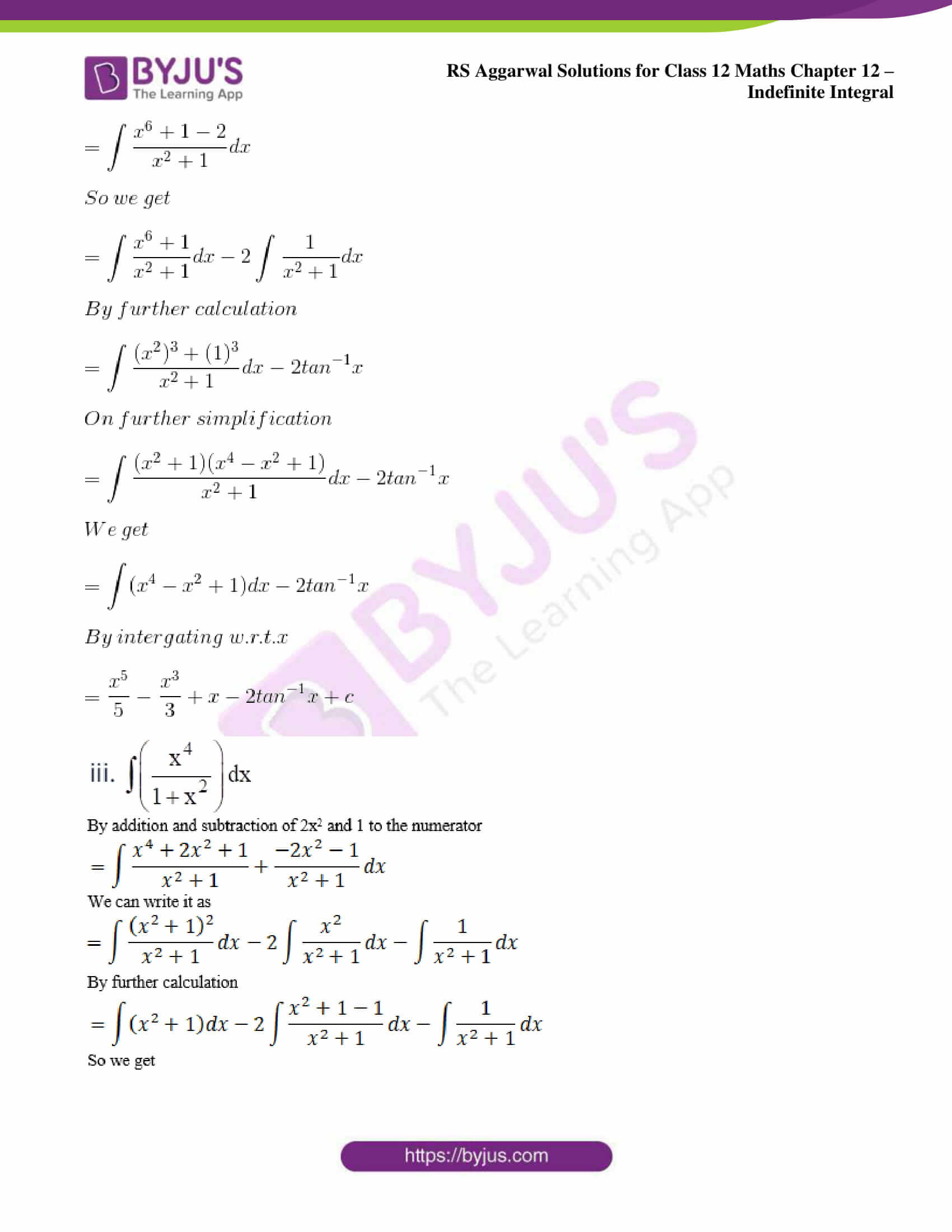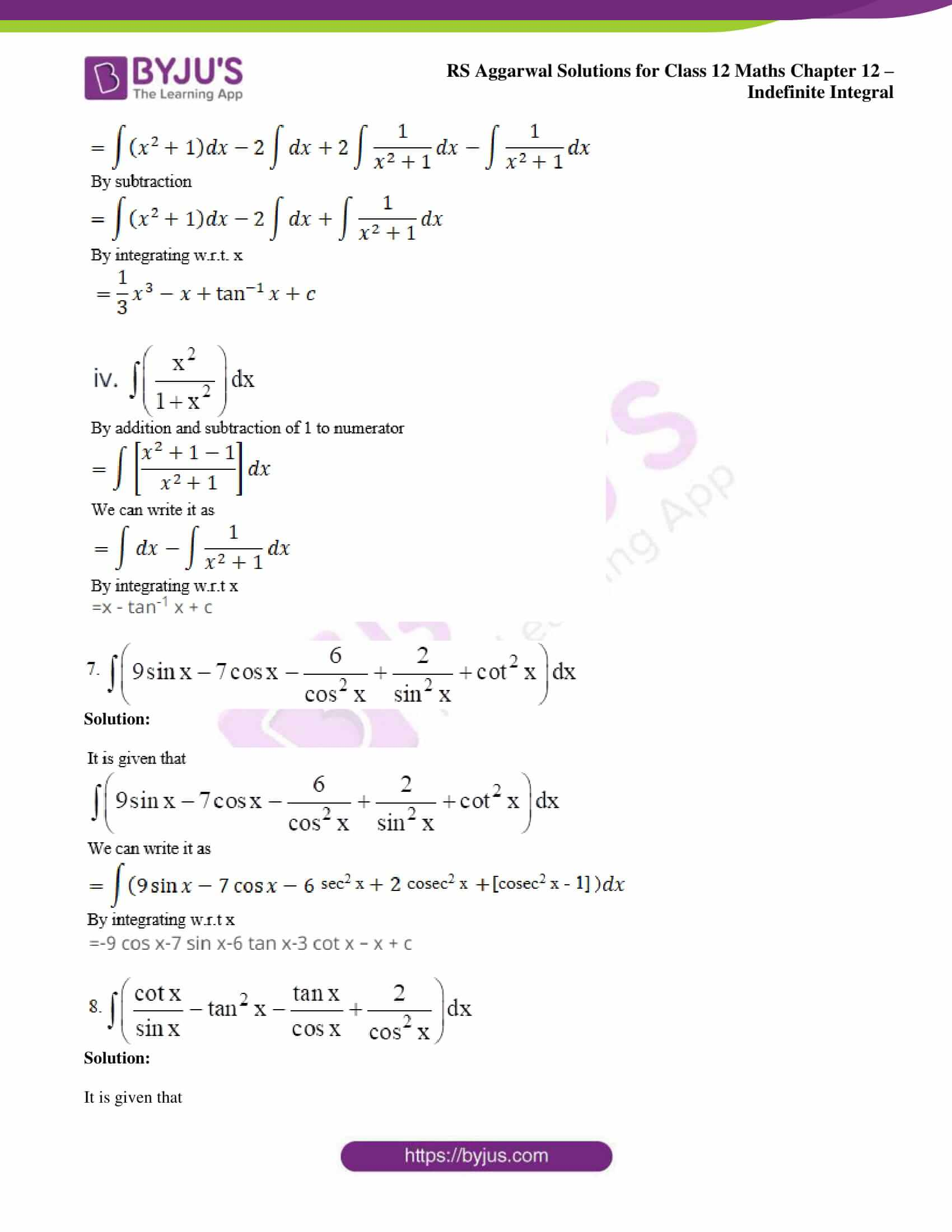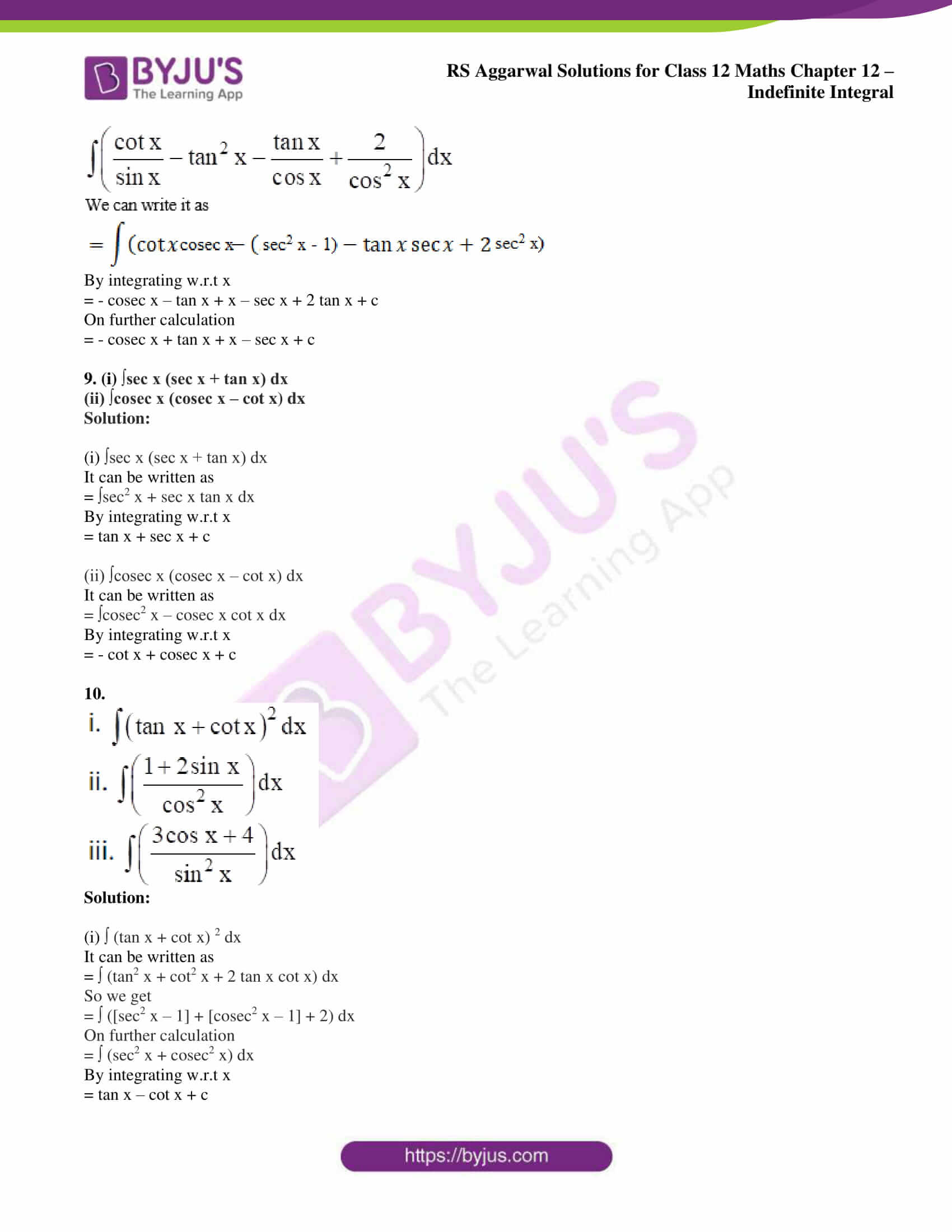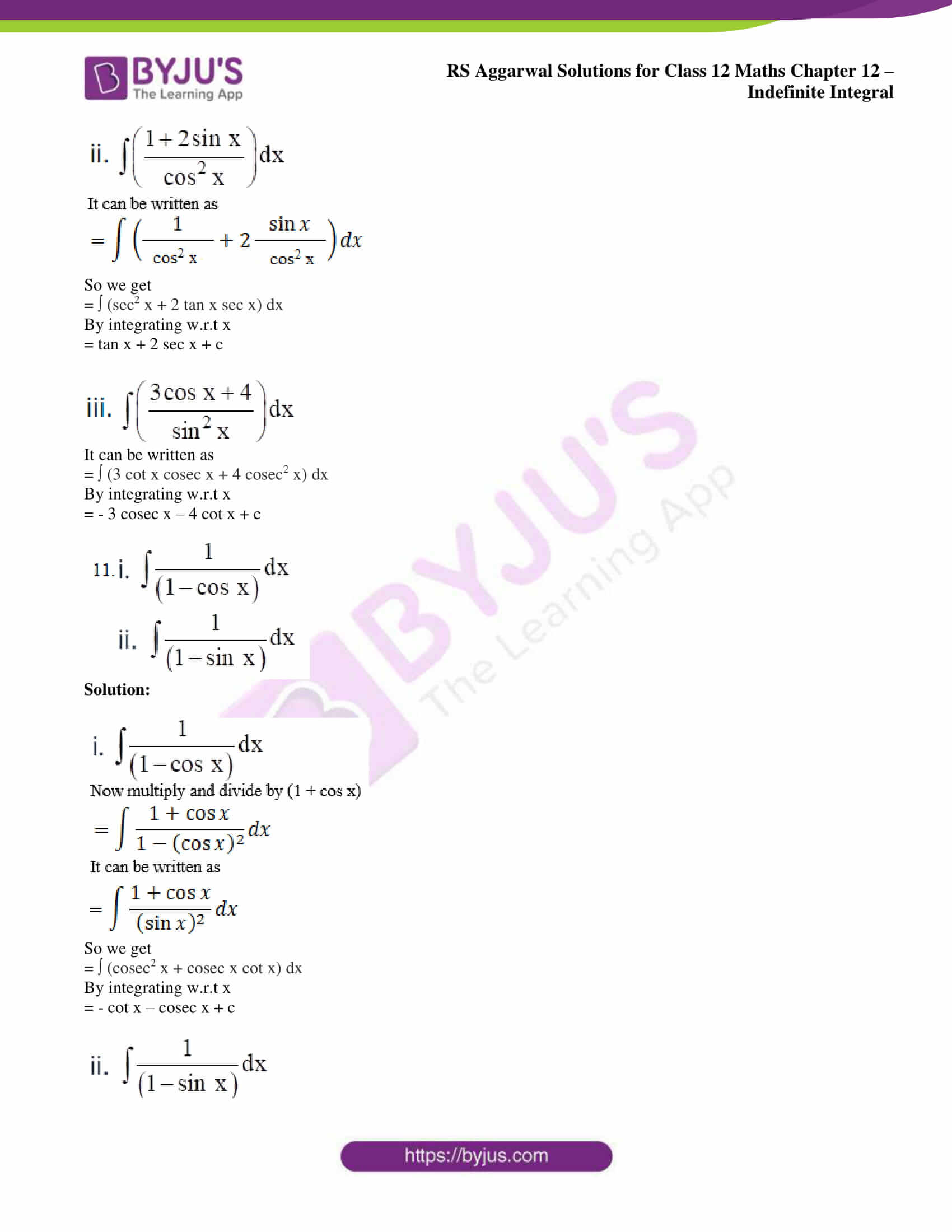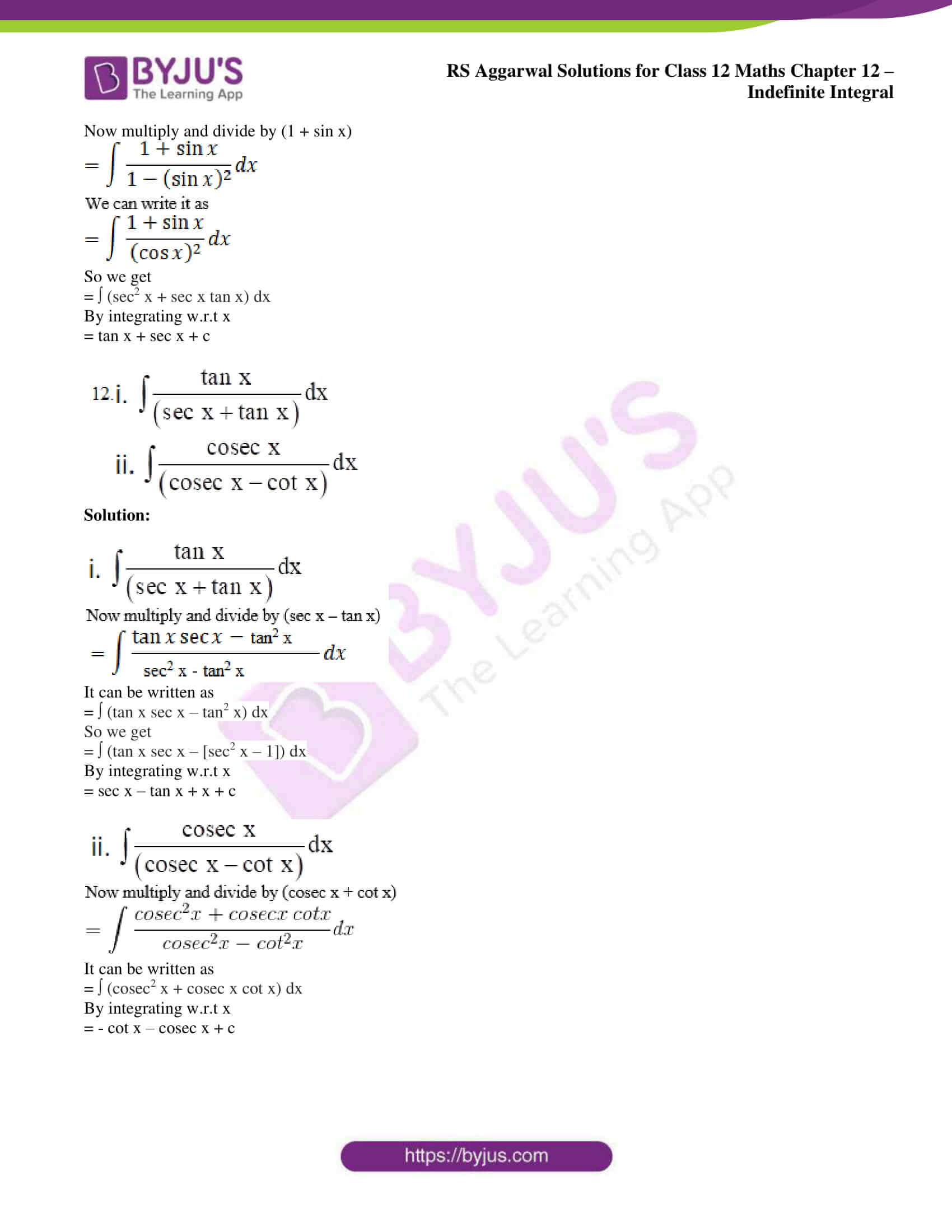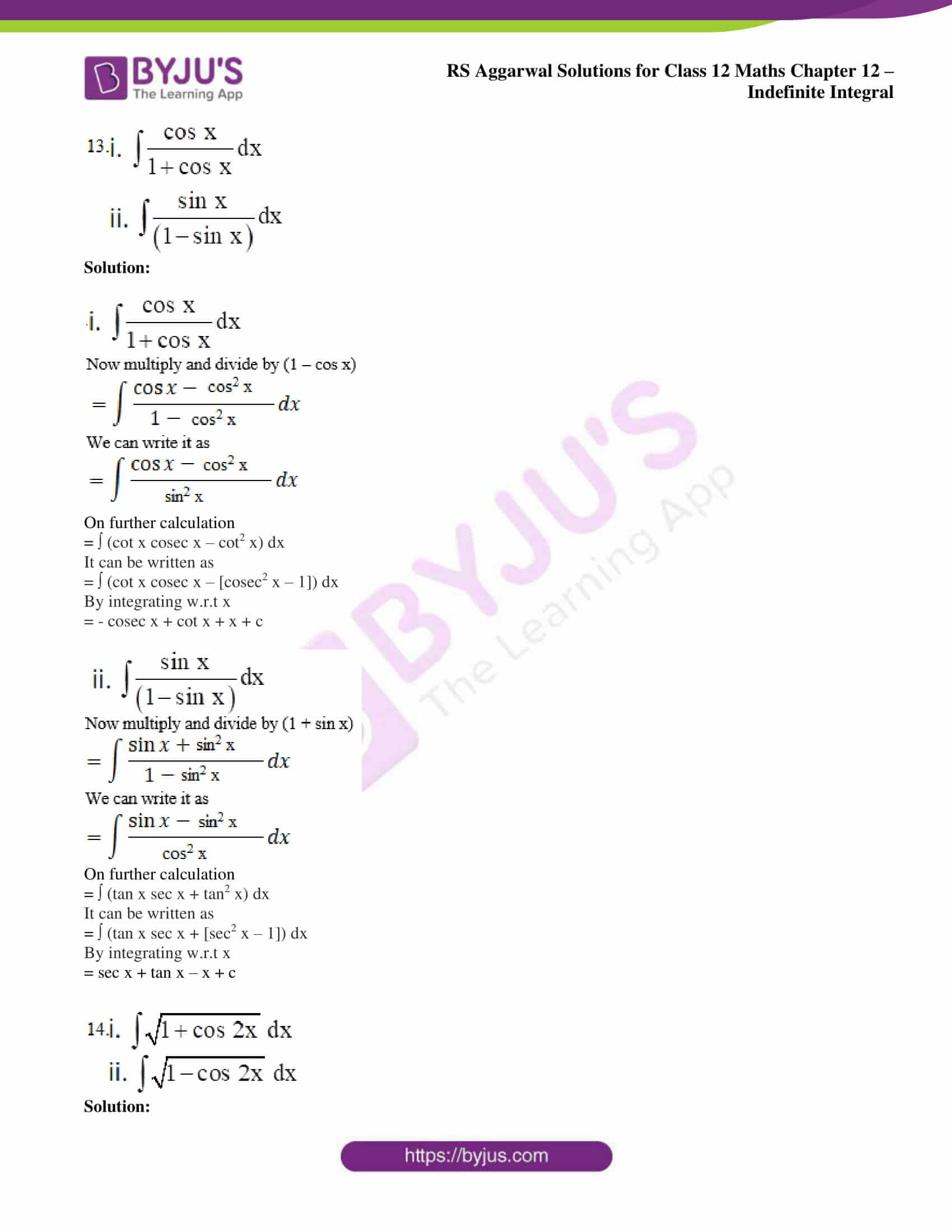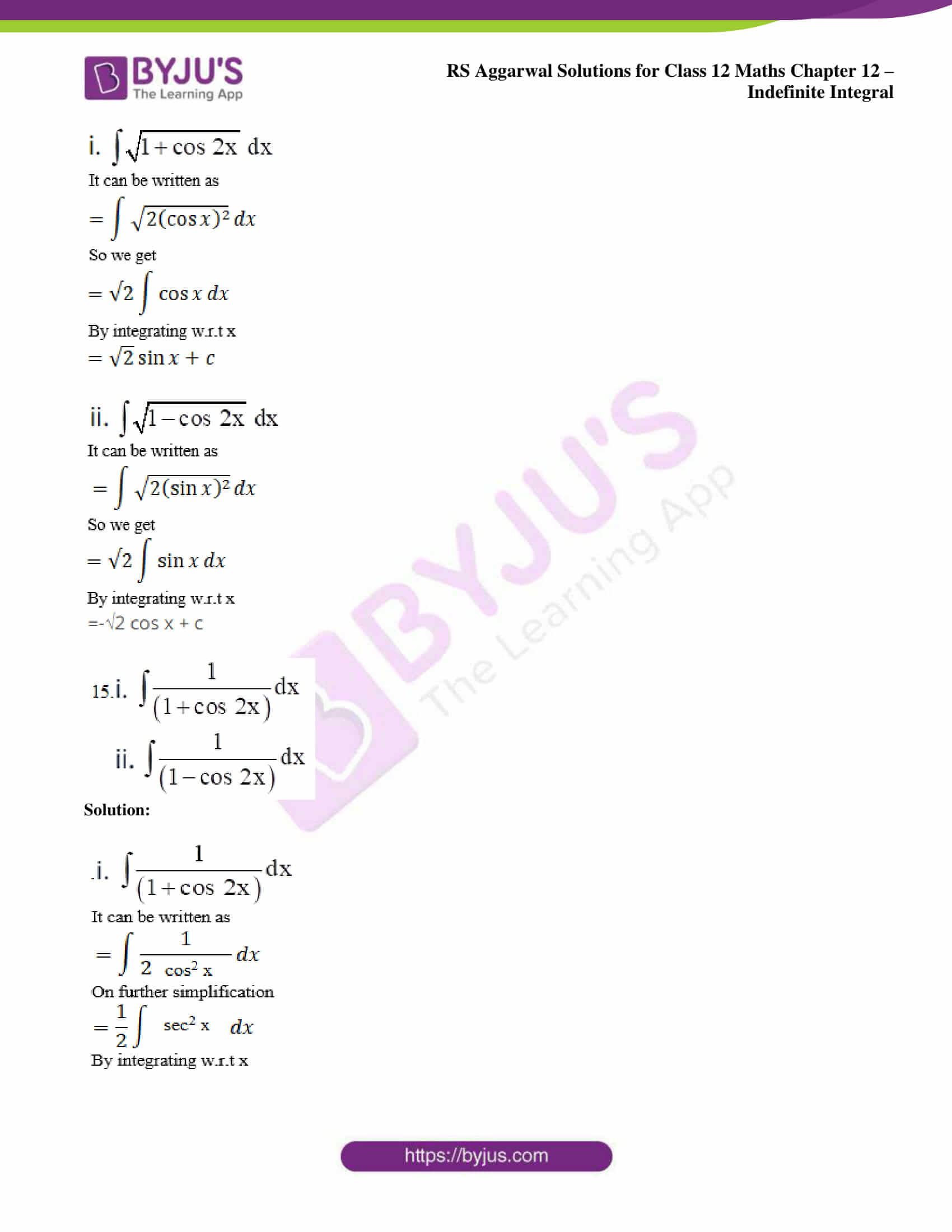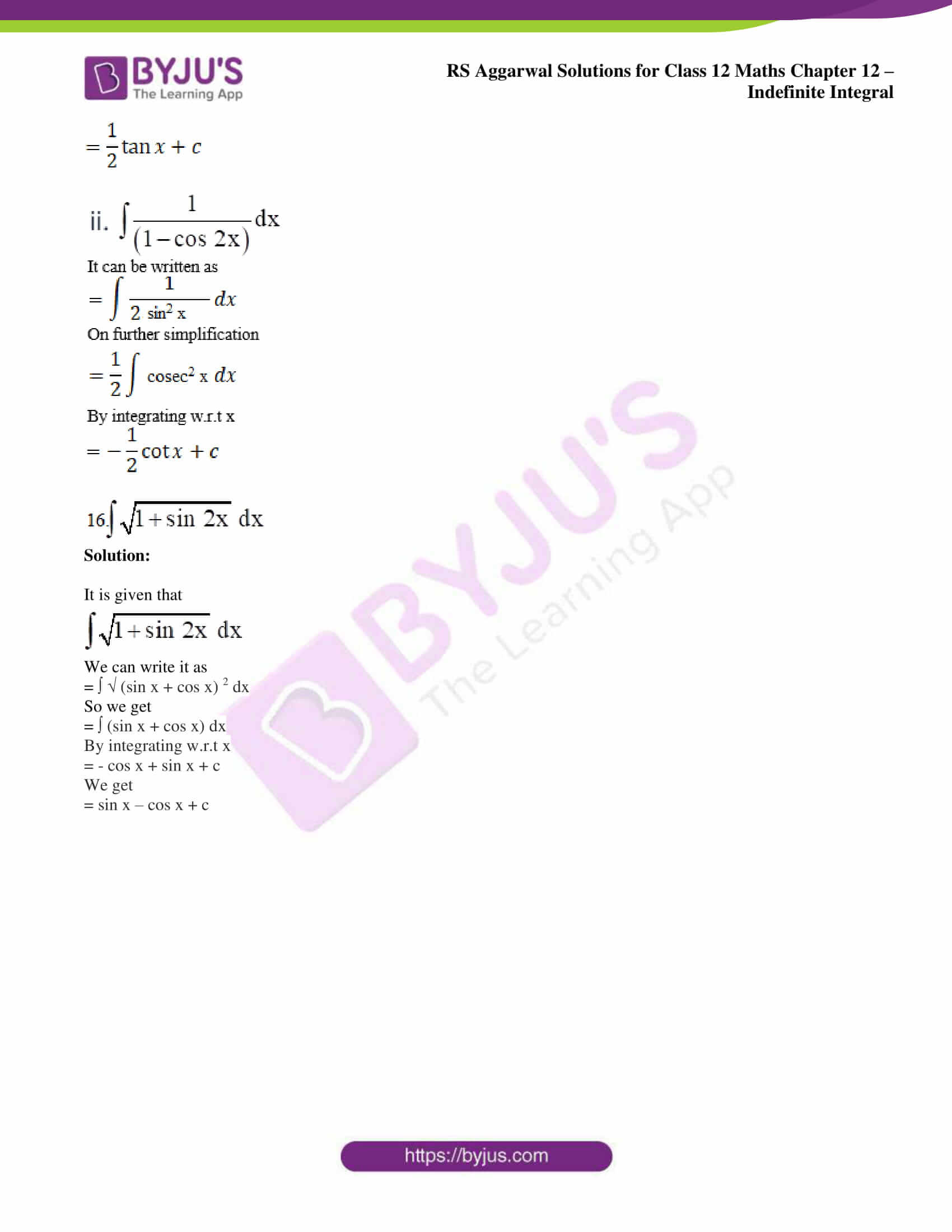## Access RS Aggarwal Solutions for Class 12 Maths Chapter 12: Indefinite Integral

Exercise 12 page: 597

Evaluate:

1.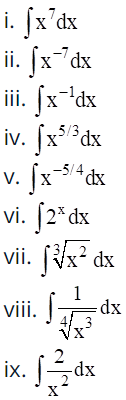Solution: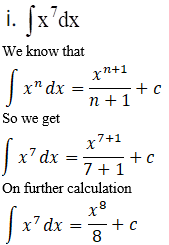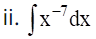We know that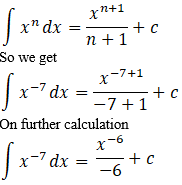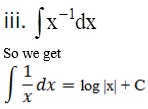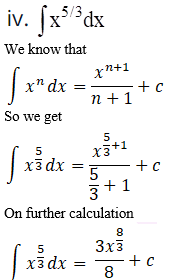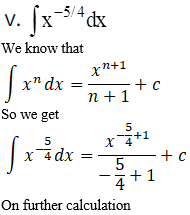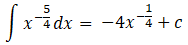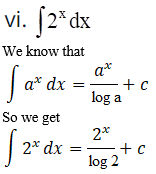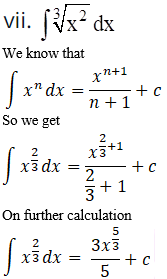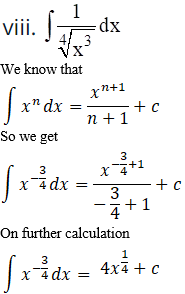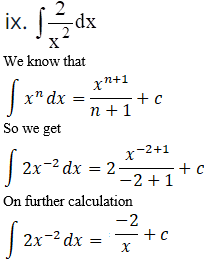2.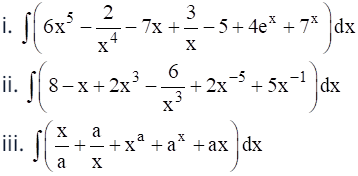Solution: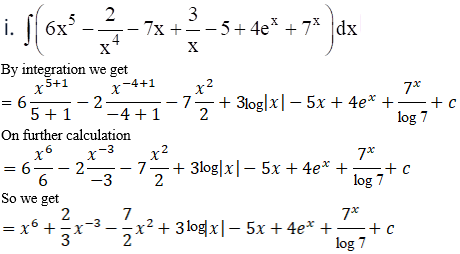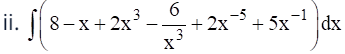By integration we get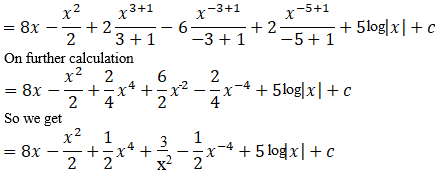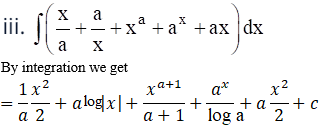3.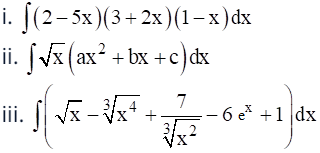Solution: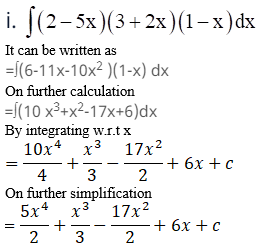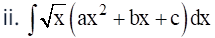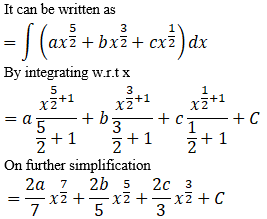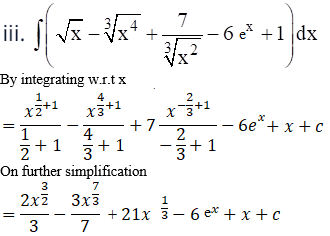4.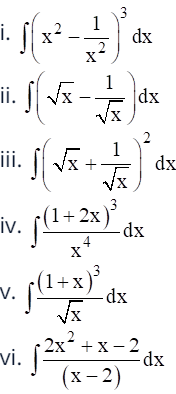Solution: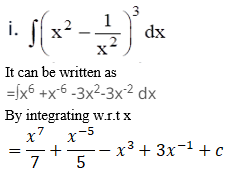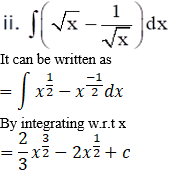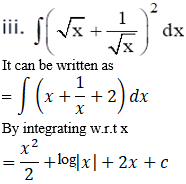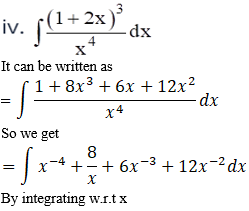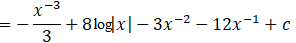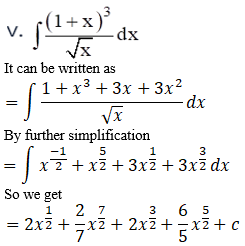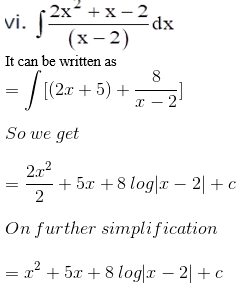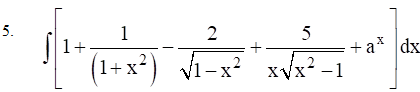Solution:

It can be written as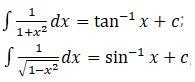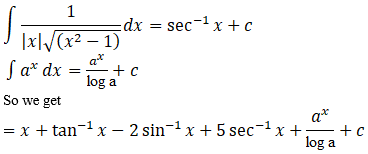6.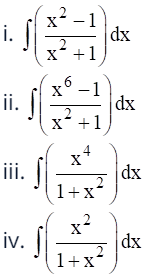Solution: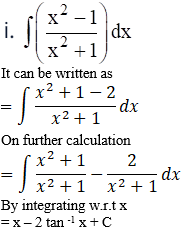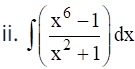It can be written as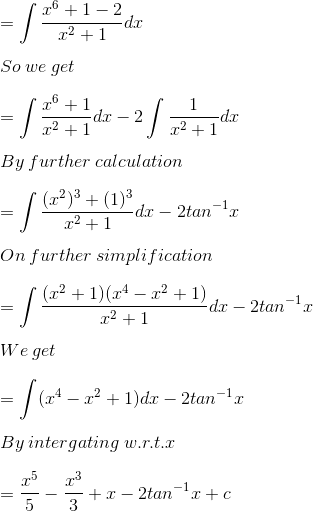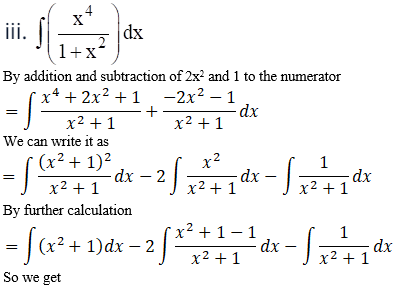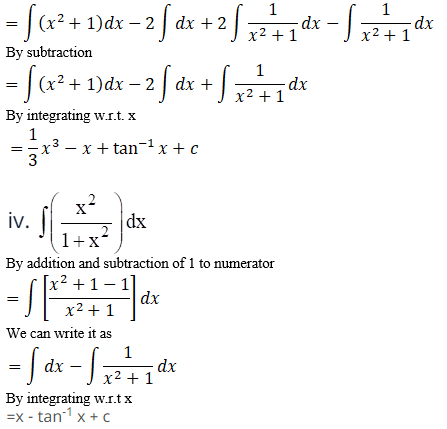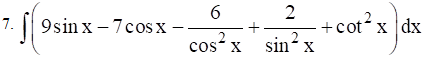Solution: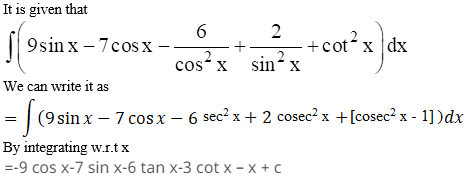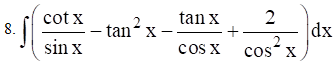Solution:

It is given that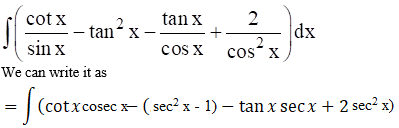By integrating w.r.t x

= – cosec x – tan x + x – sec x + 2 tan x + c

On further calculation

= – cosec x + tan x + x – sec x + c

9. (i) ∫sec x (sec x + tan x) dx

(ii) ∫cosec x (cosec x – cot x) dx

Solution:

(i) ∫sec x (sec x + tan x) dx

It can be written as

= ∫sec2 x + sec x tan x dx

By integrating w.r.t x

= tan x + sec x + c

(ii) ∫cosec x (cosec x – cot x) dx

It can be written as

= ∫cosec2 x – cosec x cot x dx

By integrating w.r.t x

= – cot x + cosec x + c

10.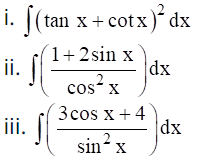Solution:

(i) ∫ (tan x + cot x) 2 dx

It can be written as

= ∫ (tan2 x + cot2 x + 2 tan x cot x) dx

So we get

= ∫ ([sec2 x – 1] + [cosec2 x – 1] + 2) dx

On further calculation

= ∫ (sec2 x + cosec2 x) dx

By integrating w.r.t x

= tan x – cot x + c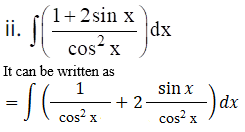So we get

= ∫ (sec2 x + 2 tan x sec x) dx

By integrating w.r.t x

= tan x + 2 sec x + c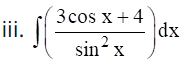It can be written as

= ∫ (3 cot x cosec x + 4 cosec2 x) dx

By integrating w.r.t x

= – 3 cosec x – 4 cot x + c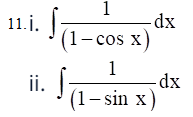Solution: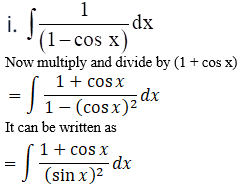So we get

= ∫ (cosec2 x + cosec x cot x) dx

By integrating w.r.t x

= – cot x – cosec x + c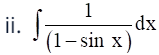Now multiply and divide by (1 + sin x)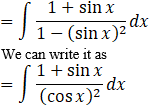So we get

= ∫ (sec2 x + sec x tan x) dx

By integrating w.r.t x

= tan x + sec x + c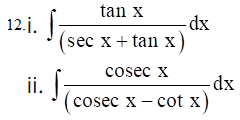Solution: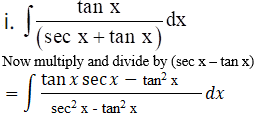It can be written as

= ∫ (tan x sec x – tan2 x) dx

So we get

= ∫ (tan x sec x – [sec2 x – 1]) dx

By integrating w.r.t x

= sec x – tan x + x + c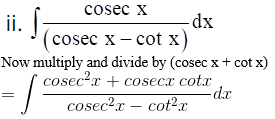It can be written as

= ∫ (cosec2 x + cosec x cot x) dx

By integrating w.r.t x

= – cot x – cosec x + c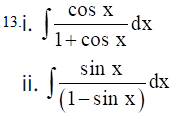Solution: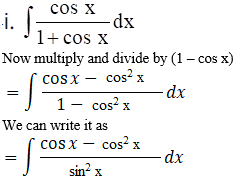On further calculation

= ∫ (cot x cosec x – cot2 x) dx

It can be written as

= ∫ (cot x cosec x – [cosec2 x – 1]) dx

By integrating w.r.t x

= – cosec x + cot x + x + c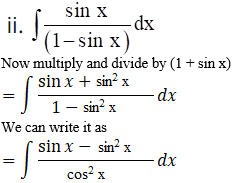On further calculation

= ∫ (tan x sec x + tan2 x) dx

It can be written as

= ∫ (tan x sec x + [sec2 x – 1]) dx

By integrating w.r.t x

= sec x + tan x – x + c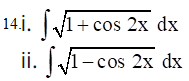Solution: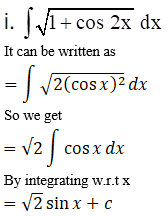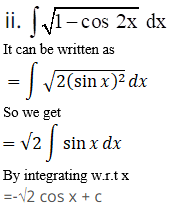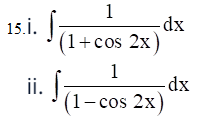Solution: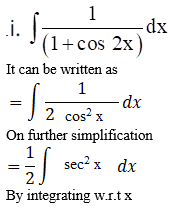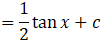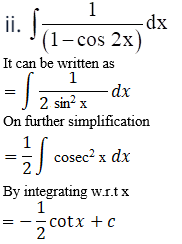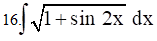Solution:

It is given that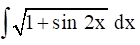We can write it as

= ∫ √ (sin x + cos x) 2 dx

So we get

= ∫ (sin x + cos x) dx

By integrating w.r.t x

= – cos x + sin x + c

We get

= sin x – cos x + c